Close
Type at least 1 character to search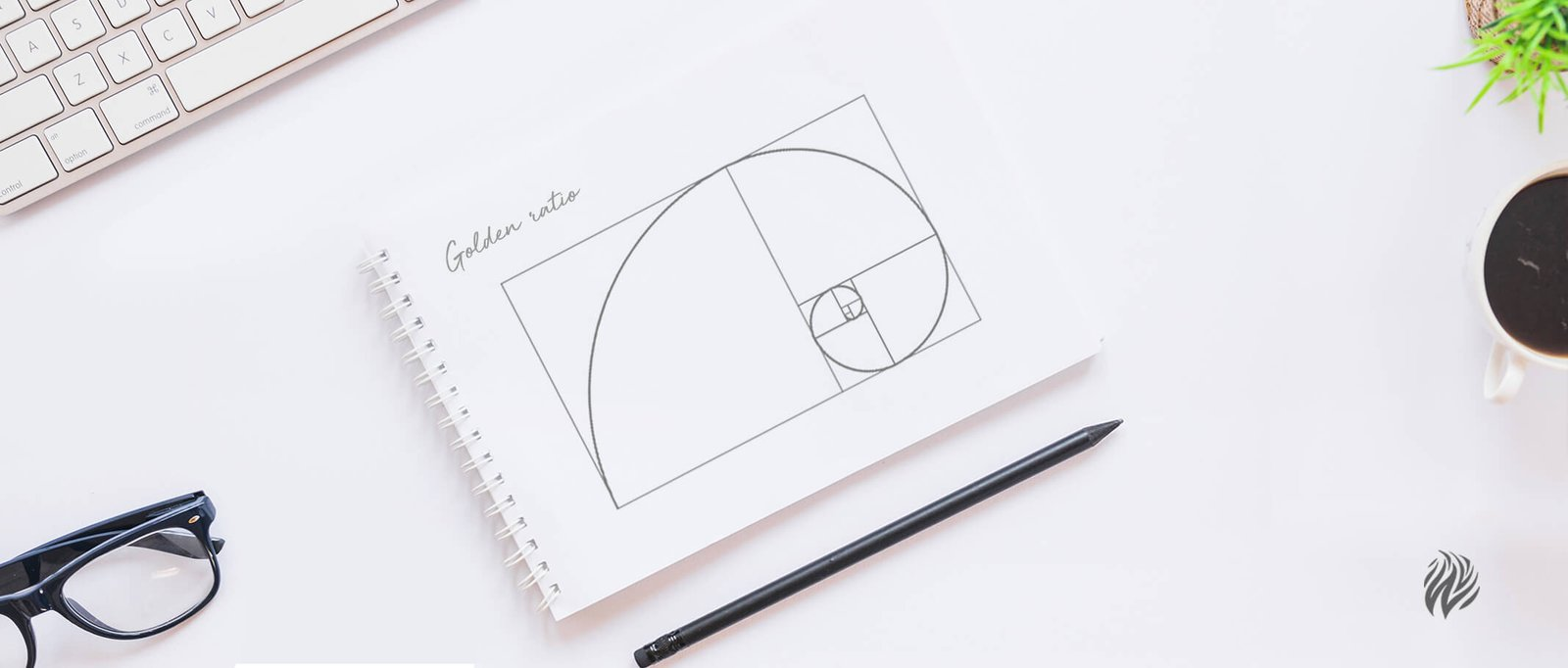Golden Ratio in Design

Would you believe this? The golden ratio is the common thing present in snail shells, plants, art, music, design, etc.

It’s known that math holds the answers to most of the general questions in this universe. Here is one of its kind. The word “Golden ratio” may give you a feel like a math lesson but this math term can be found in the real world. (which you are unaware)

By knowingly or unknowingly or designer or layman, research has found your brain mostly like to prefer artwork that follows the Golden Ratio.
This blog will first help you know about the Fibonacci series, which will help to learn about the Golden Ratio and the Golden Spiral.

What is the Fibonacci series?

The Fibonacci series is a pattern derived by adding the sum of the previous two.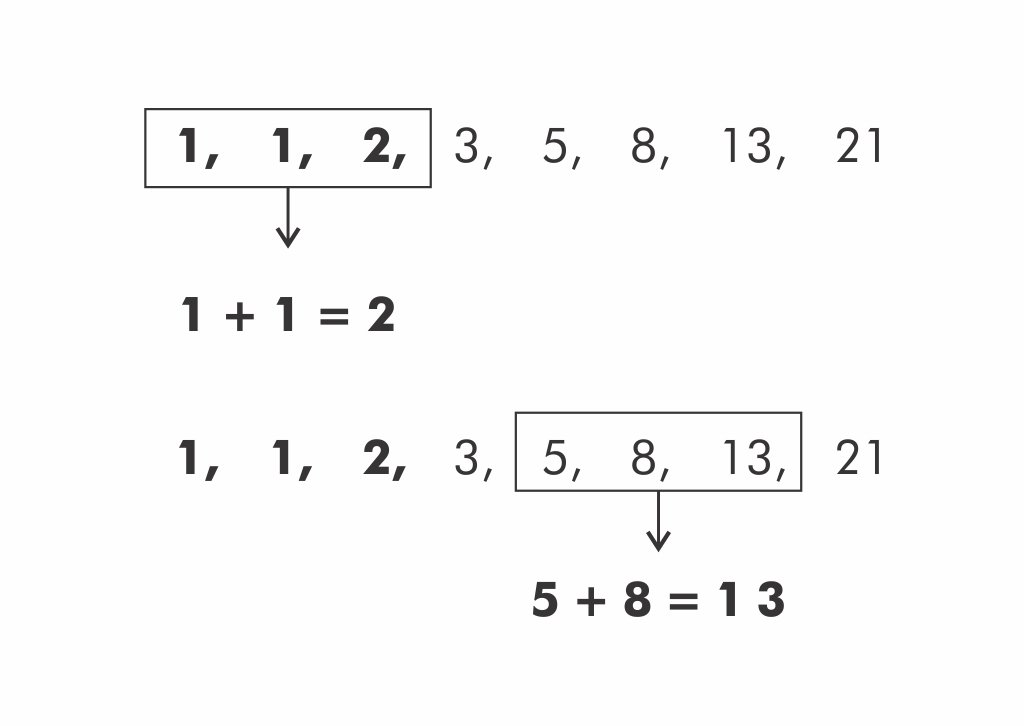The sequence will be formed as follows:

1, 1, 2, 3, 5, 8, 13, 21, 34,….so on.

What is the Golden Ratio?

The golden ratio is simply defined as the largest quantity (y) divided by the smaller quantity (x) is equal to the sum of the largest quantity (y) + smaller quantity (x) divided by (x). It should be equivalent to approximately 1.618.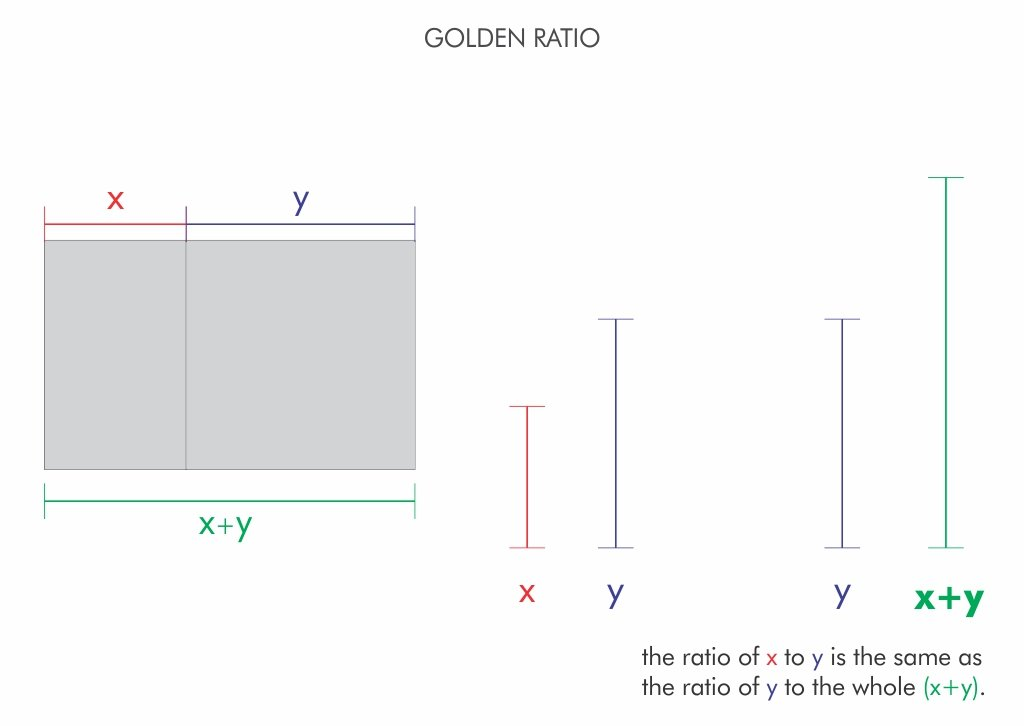The golden ratio is derived from the Fibonacci sequence, where a number represents the sum of the two preceding one.

This calculation comes handy while creating shapes, logos, layouts, and more.

What is the Golden Spiral?

When the Fibonacci series is plotted in a visual pattern, you will get a pattern like this: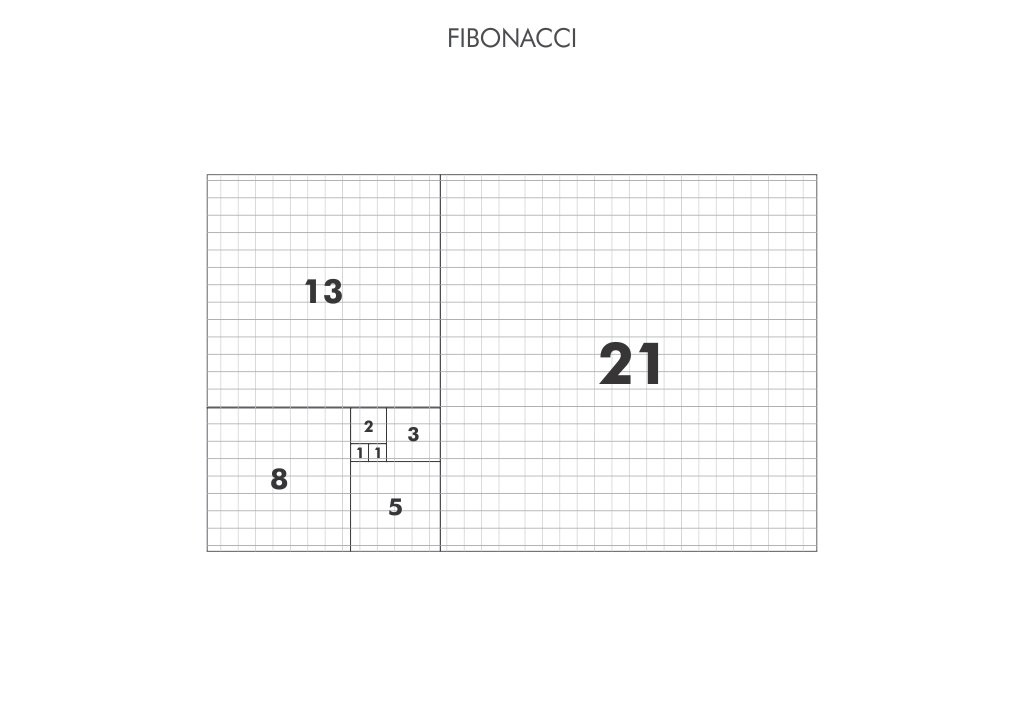When you try to connect the opposite sides of the square using an arc, it will be in a form spiral and this spiral formed using Fibonacci series is termed as the Golden Spiral.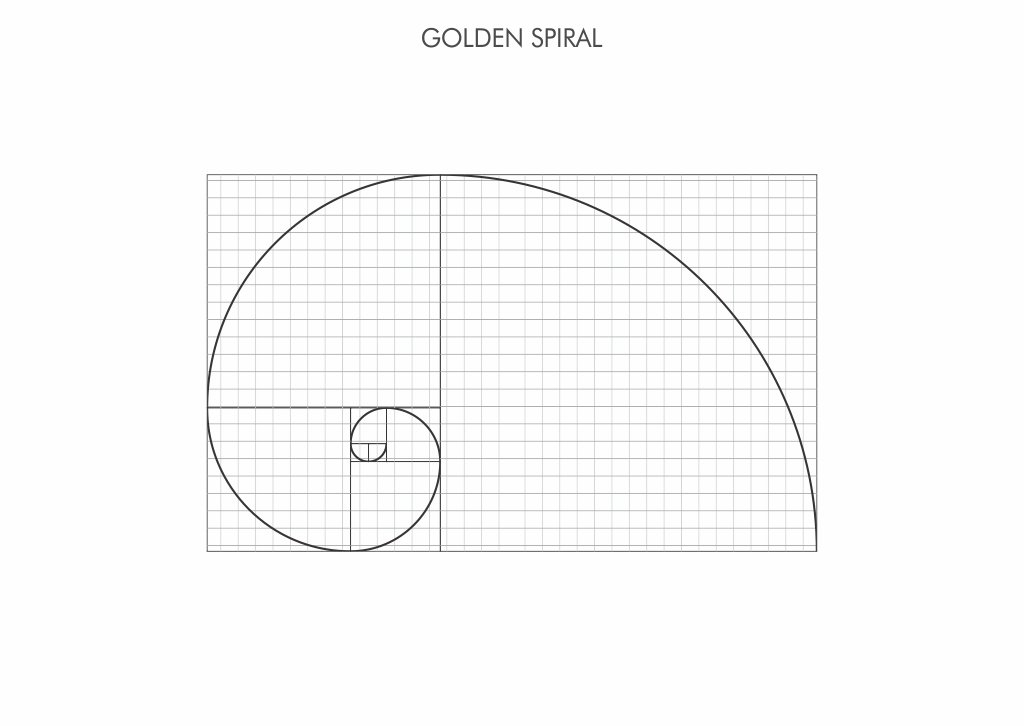Now, you know what is Fibonacci series, Golden Ratio and the Golden Spiral.
These are the fundamental thumb rules for good design and will help designers with layout, picture placement and font position.